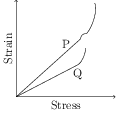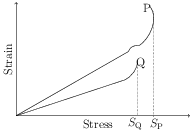# Young's Modulus

## Problems from IIT JEE

Problem (IIT JEE 2015):In plotting stress versus strain curves for two materials P and Q, a student by mistake puts strain on the $y$-axis and stress on the $x$-axis as shown in the figure. Then the correct statement(s) is(are),

1. P has more tensile strength than Q.
2. P is more ductile than Q.
3. P is more brittle than Q.
4. The Young's modulus of P is more than that of Q.

Solution:The tensile strength of a material is generally defined as the stress at which the material breaks. From the figure, P has more strength than Q (because $S_\text{P}>S_\text{Q}$). The material Q is brittle because its stress-strain curve does not show any yield. The material P is ductile as its stress-strain curve clearly show yielding (the part beyond the small kink). Thus, P is more ductile than Q. In the elastic region (straight line portion), the slope of P is more than that of Q i.e., \begin{align} \left(\frac{\text{strain}}{\text{stress}}\right)_P > \left(\frac{\text{strain}}{\text{stress}}\right)_Q &&\text{i.e.,} &&\left(\frac{\text{stress}}{\text{strain}}\right)_P < \left(\frac{\text{stress}}{\text{strain}}\right)_Q.\nonumber \end{align} Thus, Young's modulus of P is less than that of Q.